# Sea state : interpret wave patterns

In Weather4D, as generally in other applications, sea ​​state forecasts are described by ocean models. The most used is the model WW3 (Wave Watch III), published by the FNMOC (Fleet Numerical Meteorology and Oceanography Center) of the US Navy, with a Global model due 7 days and resolution 1 ° (60NM), and a European model at 3 days and resolution 0.2 ° (12NM). Meteo France publish modelsMFWAM (Wave Assimilated Model) coupled with the ARPEGE and AROME weather models with three resolutions : Global at 0.5 ° (30NM), Europe at 0.1 ° (6NM), France at 0.025 ° (1,5NM) with variable deadlines (¹).

Each of these models provides three essential data for navigation (among dozens of other oceanographic data) : the height, the direction and period waves. This is the "sea of ​​the wind" from the associated weather models.

However, these three data raise some questions : what relationship between height and period, can we predict if the waves break, what reliability when approaching the coast ? Let's try to answer it (²) …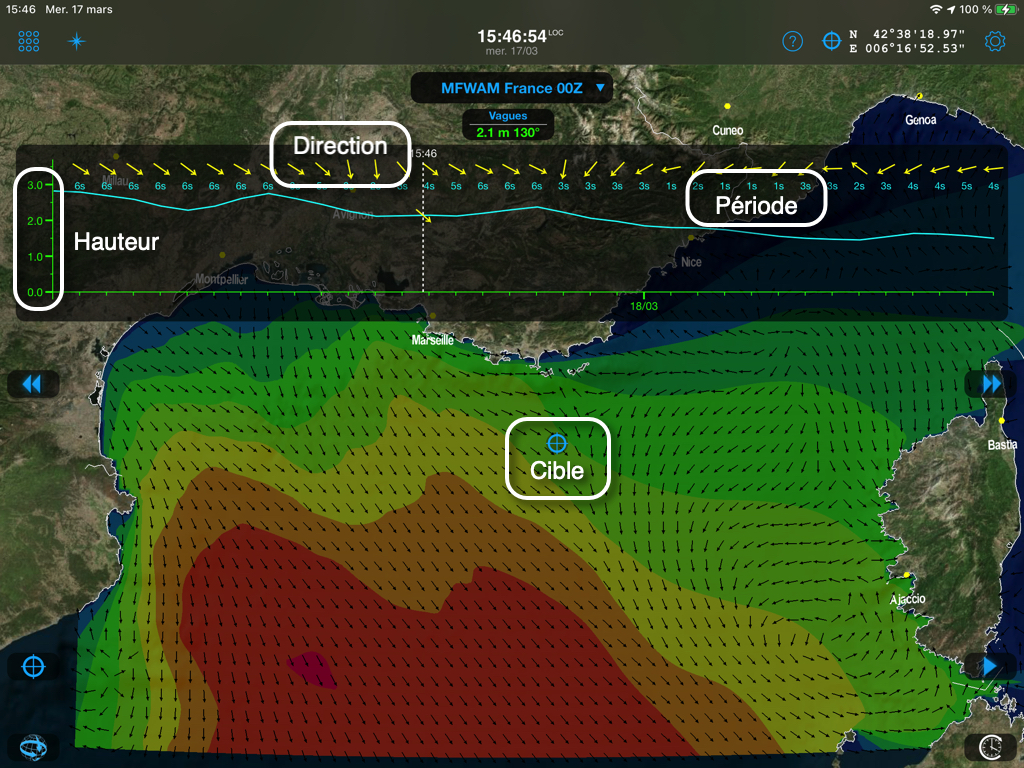The data displayed in Weather4D on the meteogram and by color fields (isoplages in steps of 0.50m).

### Wave propagation medium

We must first distinguish two wave propagation media : in deep water (dispersive environment) and shallow water (non-dispersive environment). Only the deep sea environment will be discussed here, far from the coast and the rise of the seabed.

We determine the notion of dispersive environment by a ratio between the wavelength of the waves (The) and the depth of the sea (P). Without going into mathematical details, we consider to simplify the propagation in deep water, that is, where the waves are not affected by the bottom, when P > L / 2 or L<2P, which amounts to the same. For example, with a wavelength of 150m, the depth must be greater than 75m. With a sounding at 60m, the wavelength should not exceed 120m.

### Wave characteristics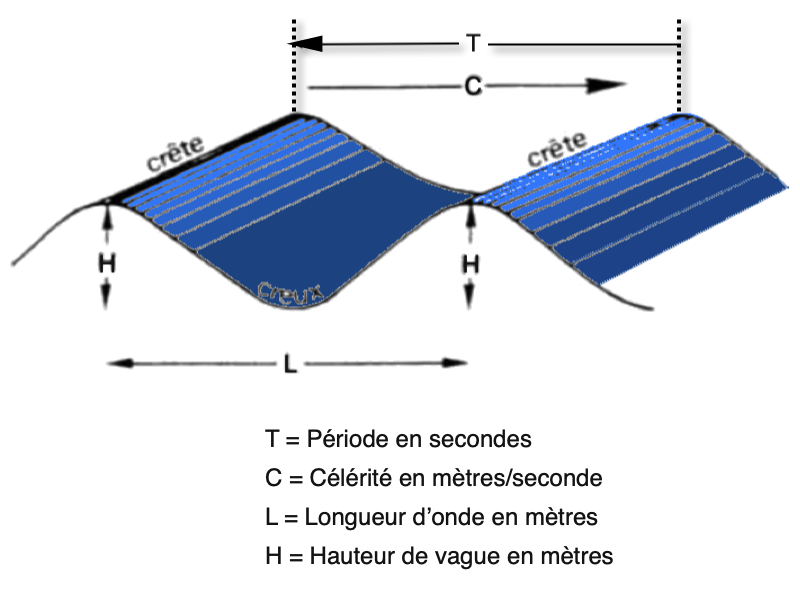The Period (T) is the time interval between two crests, or two hollows, expressed in seconds. In deep water, the Wave length (The) expressed in meters is equal to 1,5T². Example : waves of 8 seconds of period will have a wavelength of 1.5×8² = 96m (≅ 100m). The Velocity, or speed of propagation, Equals 1,5T expressed in meters per second. In the example, 1,5×8 = 12 m/s (23 knots). The Height is the vertical distance from the crest to the hollow, expressed in meters.

The values ​​of this data are closely related to the force of the wind, to the extent over which the wind blows, called generating area, and the distance over which the wind is blowing without encountering an obstacle, called the fetch.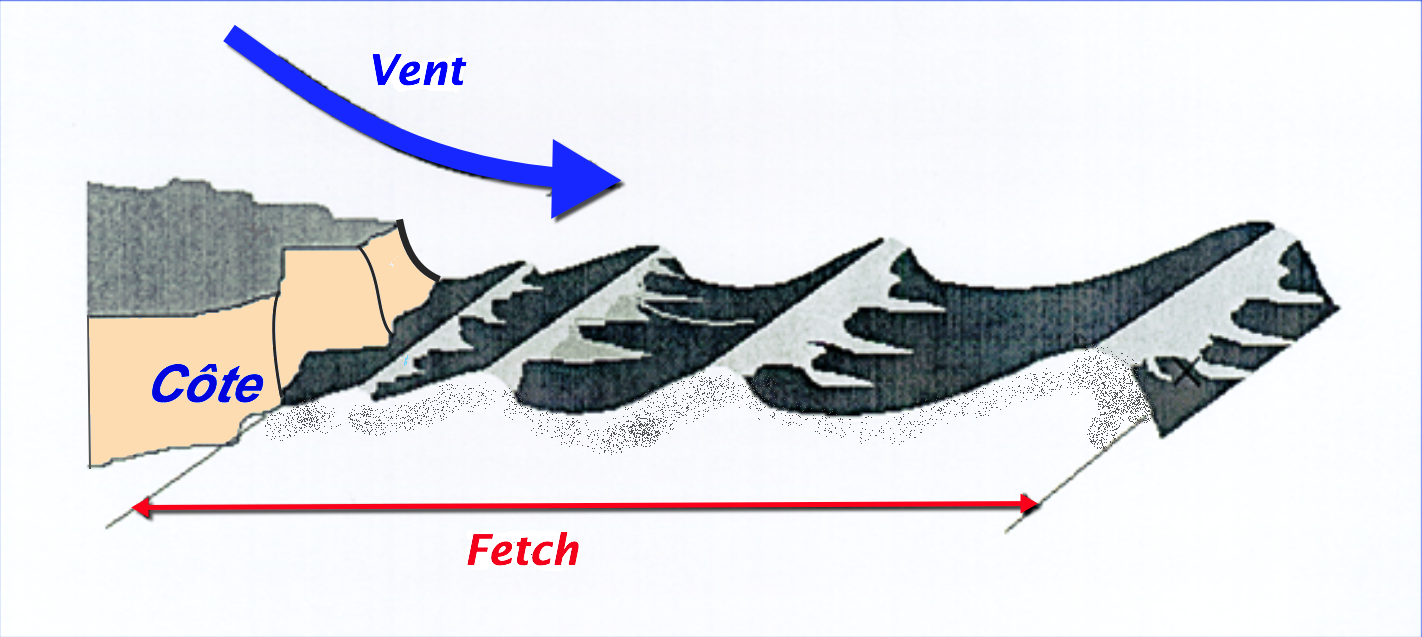The unobstructed distance over which the wind is blowing

### Wave trains

The state of the sea is often formed by the superposition of several wave systems. Groups are formed by the combination of wave trains of the same height and direction, but having slightly different periods. The waves will thus move in groups whose speed in deep water will be about half the speed of each isolated wave.. The speed of the group is therefore calculated V = C/2, soit V = 1.5T / 2 = 0,75T. Example : the speed of a group of waves whose period is 8 seconds will be 1.5×8/2 = 6m / s (12 knots). We therefore cyclically observe groups of steeper waves, the heights being added, and much lower wave intervals.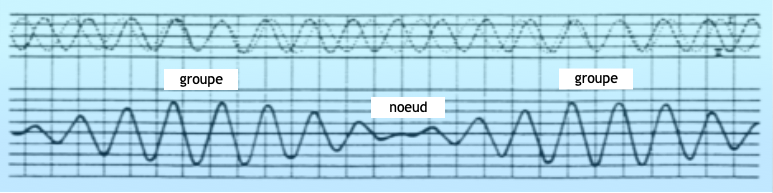The groups of waves are formed by the shift of the periods which creates cyclical juxtapositions (²)

### The surge

The breaking of a wave is determined by the notion ofCamber (γ). This data is defined by the formula γ = H / L, or the ratio of the height of the waves to the wavelength. We prove by calculation that the wave is breaking, in deep water, when the camber γ>0,14. This limit value can be reached, either by the force of the wind alone, either by the wind against a strong current (in the Channel, for example, when the tide reverses against the wind), by crossing trains of waves of different directions, or by a rising sea floor (in the latter case we enter a non-dispersive environment).

Example with waves of H = 5m and T = 6s, limited breaking risk :

• L = 1,5T² =1,5×6² = 54m
• γ = H/L = 5/54 = 0,09

We can easily calculate, for each wave height, the limit period giving a camberγ>0,14. here are some examples :

 Height Calculated period Rounded period 5meters 4,88s. 5s. 6meters 5,35s. 5,5s. 8meters 6,2s. 6,5s. 10meters 6,9s. 7s.

But beware, do not confuse "breaking wave" and "breaking crest" which occurs much earlier, from 30-35 knots of wind, when the gusts sweep the tops of the waves as in the illustration below. On the other hand, in the groups of waves mentioned above, at the same time, the groups of more abrupt waves can reach heights causing a limit camber.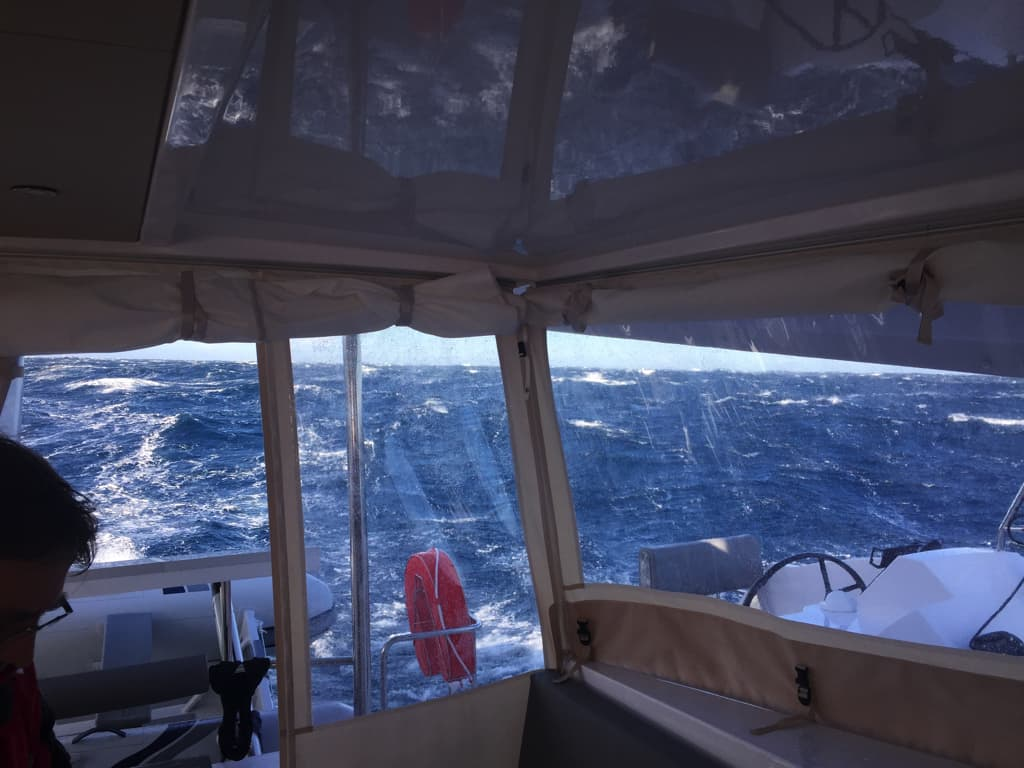Crests breaking under a Tramontana at 40 established knots, approaching Cap Sicié.

Approaching the coast, things get complicated. Wave calculations in a non-dispersive environment, therefore by shallow depth, become more complex and are the subject of a large literature of specialists, i don't belong. Each of us is well aware that the rise of the continental shelf causes a significant worsening of the sea state in strong westerly winds., even more on the English Channel when the tide is opposite, and that the Tramontana can form a breaking swell on the northern coasts of the Balearic Islands, as in the following illustration.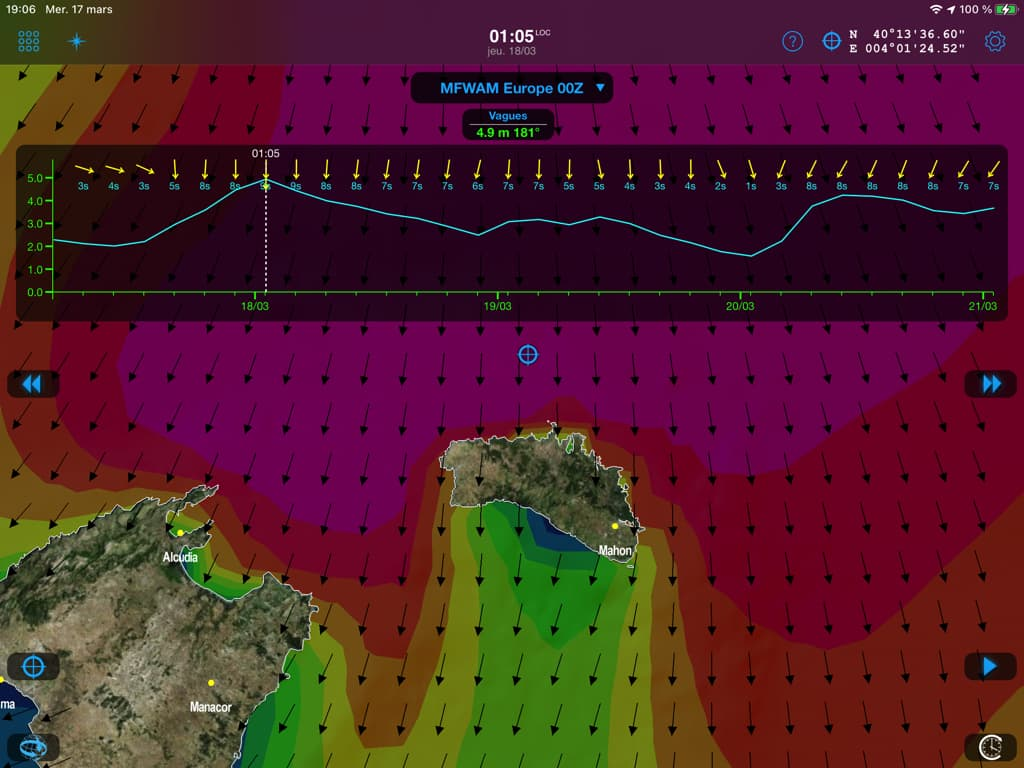The sea of ​​L = 100m will rise on the sounding line 50m north of Menorca and break to the coast.

Such a situation makes surfers happy !

–––
1.Pajot wrote: